# The Sloshing Problem.¶

By Jonas Tjemsland, Andreas Krogen, Håkon Ånes and Jon Andreas Støvneng.

Last edited: 2016-03-13

This Notebook is based on an assignment given in the subject TMA4320 Introduksjon til vitenskapelige beregninger at NTNU . The assignment was prepared by Jon Vegard Venås and Anton Evgrafov. The codes are based on the answers by Gjert Magne Knutsen, Daniel Halvorsen and Jonas Tjemsland.

In this Notebook we are going to study a mathematical model for a harmonic motion of the free surface of a fluid in two dimensional containers. The numerical approach is based on a linear model, which reduces the problem to a Steklov eigenvalue prblem (see the appendices below). The problem is analyzed using source points and collocation points.

The problem analyzed is often known as the sloshing problem. The concepts introduced in this Notebook has a number of important applications, such as transportation of fluids in large containers on ships, and many everyday analogies, such as spilling from a coffee cup.

In :
# Import libraries
import numpy as np
from scipy import linalg as la
import matplotlib
import matplotlib.pyplot as plt
from IPython.core.display import HTML
%matplotlib inline

# We are going to get some errors from casting imaginary numbers to real numbers,
# because of numerical rounding errors, and divisions by zero in la.eig(). We
# therefore disable warning messages.
import warnings
warnings.filterwarnings('ignore')

# Set common figure parameters
newparams = {'axes.labelsize': 8, 'axes.linewidth': 1, 'savefig.dpi': 200,
'lines.linewidth': 1, 'figure.figsize': (8, 3),
'ytick.labelsize': 7, 'xtick.labelsize': 7,
'legend.fontsize': 7, 'legend.frameon': True,
'legend.handlelength': 1.5, 'axes.titlesize': 7,}
plt.rcParams.update(newparams)


## 1 Introduction.¶

We are going to study a mathematical model describing a harmonic motion of a fluid $\Omega$ in a container $\Gamma_\text{B}\subset \partial \Omega$ with a free surface $\Gamma_\text{F}\subset \partial \Omega$. For simplicity, we are going to consider this problem in two dimensions \begin{align} \Delta \psi(x,y) &= 0,\quad(x,y) \in \Omega,\\ \boldsymbol{n}\cdot\nabla \psi(x,y) &= 0, \quad(x,y) \in \Gamma_\text B,\\ \qquad\;\boldsymbol{n}\cdot\nabla \psi(x,y) &= \lambda\psi(x,y),\quad(x,y) \in \Gamma_\text F,\\ \end{align} where $\boldsymbol{n}=[n_x,n_y]$ is the unit vector pointing outwards of of the domain $\Omega$ (and $\Delta=\nabla^2$ is the Laplace operator). This problem is known as the mixed Steklov eigenvalue problem for the Laplace operator. Physically, this can be interpreted as a container which is infinitely long in $z$-direction (into the plane of the screen). The setup is shown in the picture below.For an arbitrary $\lambda$, the problem has the trivial solution $\psi=0$. The $\lambda$-values that yields at least one non-trivial solution, are called eigenvalues of the problem, and the corresponding solutions $\psi$ are called eigenfunctions. The eigenvalues $\lambda>0$ are related to the resonance frequencies of the fluid in the container, and the eigenfunctions $\psi$ on $\Gamma_\text{F}$ gives the form of the waves on the free surface, \begin{equation} h(x,t)=\frac{\omega}{g}\sin(\omega t)\psi(x,H), \end{equation} where $g$ is the gravitational acceleration and $\omega=\sqrt{g\lambda}$ is the wave frequency. This relation and the Steklov eigenvalue problem are discussed in the appendices below. In this Notebook we are going to normalize the the waves such that the amplitude becomes 1.

## 2 Representation of numerical solutions.¶

Let us define the function $\Psi:\mathbb{R}\backslash\{\boldsymbol 0\}\to \mathbb R$ as \begin{equation} \Psi(x,y) = \log \sqrt{x^2+y^2}. \end{equation} For a given number of source points $(x_1^s,y_1^s),...,(x_N^s,y_N^s) \in \mathbb R^2 \backslash(\Omega\cup \partial \Omega)$ we define the function \begin{equation} \psi(x,y) = \sum_{j=1}^N \alpha_j \Psi(x-x_j^s,y-y_j^s), \end{equation} where $\alpha_j$ are unknown coefficients we want to calculate. This function is a solution of the first equation of the mixed Steklov eigenvalue problem (Poisson's equation) for arbitrary constants $\alpha_1,...,\alpha_N$.

Our goal is therefore to find $\alpha_1,...,\alpha_N$ such that the two last equations are approximately fulfilled.

## 3 Numerical solution of the problem.¶

We are going to place $N$ (approximately) uniformly distributed data point on the boundary $\partial \Omega$ and require that the two last equation of the problem are true in these points. We then hope that the equations are approximated on the whole boundary. These points are so-called collocation points. Let $N_\text B$ be the number of collocation points $(x_1^c,y_1^c),...,(x_{N_\text B}^c,y_{N_\text B}^c)$ on $\Gamma_\text B$ and $N_\text F$ the number of collocation points $(x_{N_{\text B}+1}^c,y_{N_{\text B}+1}^c),...,(x_{N_\text B+N_\text F}^c,y_{N_\text B+N_\text F}^c)$ on $\Gamma_\text F$. For each collocation point along the boundary $\Gamma_\text B$ and $\Gamma_\text F$ one can use the second and the third equation respectively to derive equations with $\alpha_j$ as unknowns. This will result in $N=N_\text B+N_\text F$ equations and unknowns.

To simplify our numerical calculations we are going to represent this system of equations as the matrix form $A\boldsymbol \alpha = \lambda B \boldsymbol \alpha$, where $\boldsymbol \alpha = [\alpha_1,...,\alpha_N]^\text T$. If we insert $\psi(x,y)$ into the Steklov eigenvalue problem, we find that $A$ and $B$ are $N\times N$ matrices with the entries $$A_{ij}=\frac{n_x(x_i^c-x_j^s)+n_y(y_i^c-y_j^s)}{(x_i^c-x_j^s)^2+(y_i^c-y_j^s)^2},$$ $$B_{ij}=\begin{cases} \log\sqrt{(x_i^c-x_j^s)^2+(y_i^c-y_j^s)^2}, &i=N_{\text{B}+1},...,N,\\ 0, &\text{otherwise.} \end{cases}$$

A non-trivial solution $\boldsymbol \alpha \neq 0$ to the system of equations $(A-\lambda B)\boldsymbol \alpha = 0$ exists only if the matrix $A-\lambda B$ is singular. In this case we call the solution $(\lambda,\boldsymbol \alpha)$ an eigenvalue/eigenvector couple to a generalized eigenvalue problem given by the matrices $(A,B)$. This is easily solved by using the function eig() defined in the python package scipy.linalg.

## 4 Representaion of geometry and deciding source- and collocation points.¶

We are going to represent the boundary as the parametrizations \begin{align} \Gamma_\text B & = \{\boldsymbol C_\text B(\xi):0\leq \xi \leq \Xi_\text B\},\\ \Gamma_\text F & = \{\boldsymbol C_\text F(\xi):0\leq \xi \leq \Xi_\text F\}, \end{align} where $\boldsymbol C_\text B$ and $\boldsymbol C_\text F$ are piecewise differentiable functions. We are going to choose the sourcepoints a length $\delta>0$ from the collocation points outwards from det container.

### 4.1 Box geometry¶

A two dimensional box of length $L$ and height $H$ can be parameterized by $$\boldsymbol C_\text B(\xi)=\begin{cases} \boldsymbol e_y(H-\xi),\qquad\qquad\qquad 0\leq \xi<H,\\ \boldsymbol e_x(\xi-H), \qquad\qquad\qquad\! H\leq xi<H+L,\\ L\boldsymbol e_x + \boldsymbol e_y (\xi -L-H), \qquad H+L\leq\xi\leq 2H+L=\Xi_\text B, \end{cases}$$ $$\boldsymbol C_\text F(\xi) = \boldsymbol e_x(L-\xi)+H\boldsymbol e_y, \qquad\qquad 0\leq \xi \leq L = \Xi_\text F.$$ We now know enough to create a function that calculates the source and collocation points of the box geometry. Note that the collocation points should not be placed too close to the corners, where $\Gamma_\text B$ and $\Gamma_\text F$ are discontinuous.

In :
def csPointsBox(N,L,H,delta):
""" Calculates the source points and the collocation points of the box geometry.
The points are chosen uniformly on each side, such that the total number of points
are even.
Parameters:
N:     int. Number of source and collocation points.
L:     float. Length of the box.
H:     float. Height of the box.
delta: float. Distance between the source and collocation points.
Returns:
xc:    float arr. 2xN array of the collocation points on the container.
xs:    float arr. 2xN array of the corresponding source points.
"""
# Checks if N is even. If not, add one.
if (N % 2):
N = N + 1

# Number of source and collocation points on the different sides. N=2*NL+2*NH.
NH = int(round(N*H/(2*L + 2*H))) # On the vertical lines.
NL = int(N/2 - NH) # On the horizontal lines.

# Spacing between the source and collocation points.
spacingL = L/NL
spacingH = H/NH

# The unit normal vector of the container.
n = np.zeros((2,N))
n[0,0:NH] = -np.ones(NH)
n[0,NH + NL:2*NH + NL] = np.ones(NH)
n[1,NH:NH + NL] = -np.ones(NL)
n[1,2*NH + NL:N] = np.ones(NL)

# Calculates the collocation points, and saves them in a 2xN matrix.
xc = np.zeros((2,N))
xc[0,NH:NH + NL] = np.arange(spacingL/2.0,L,spacingL)
xc[0,NH + NL:2*NH + NL] = L*np.ones(NH)
xc[0,2*NH + NL:N] = np.arange(spacingL/2.0,L,spacingL)
xc[1,0:NH] = np.arange(H-spacingH/2.0,0.0,-spacingH)
xc[1,NH + NL:2*NH + NL] = np.arange(spacingH/2,H,spacingH)
xc[1,2*NH + NL:N] = H*np.ones(NL)

# Calculates the source points, and saves them in a 2xN matrix.
xs = xc + delta*n

return xc, xs


The following code visualizes the source points and the geometry. To get good results for the final solution, one may have to choose different $N$ and $\delta$.

In :
L = 3.0 # Length of the box.
H = 4.0 # Height of the box.
N = 40 # Number of collocation and source points.
delta = 0.4 # Distance between the source and collocation points.

# Calculates the collocation points and the source points.
xc, xs = csPointsBox(N,L,H,delta)

# Visualize the results.
# Plotting the container and the fluid surface.
plt.plot([0,0,L,L],[H,0,0,H],'k',label=r'$\Gamma_B$'); # The container.
plt.plot([0,L],[H,H],'b',label=r'$\Gamma_F$'); # The fluid surface.
# Plotting collocation and source points.
plt.plot(xc,xc,'r*',label='Collocation points')
plt.plot(xs,xs,'g*',label='Source points')
# Figure texts.
plt.title('Collocation points and source points for the box geometry.')
plt.xlabel('x'), plt.ylabel('y'), plt.axis('equal'), plt.legend();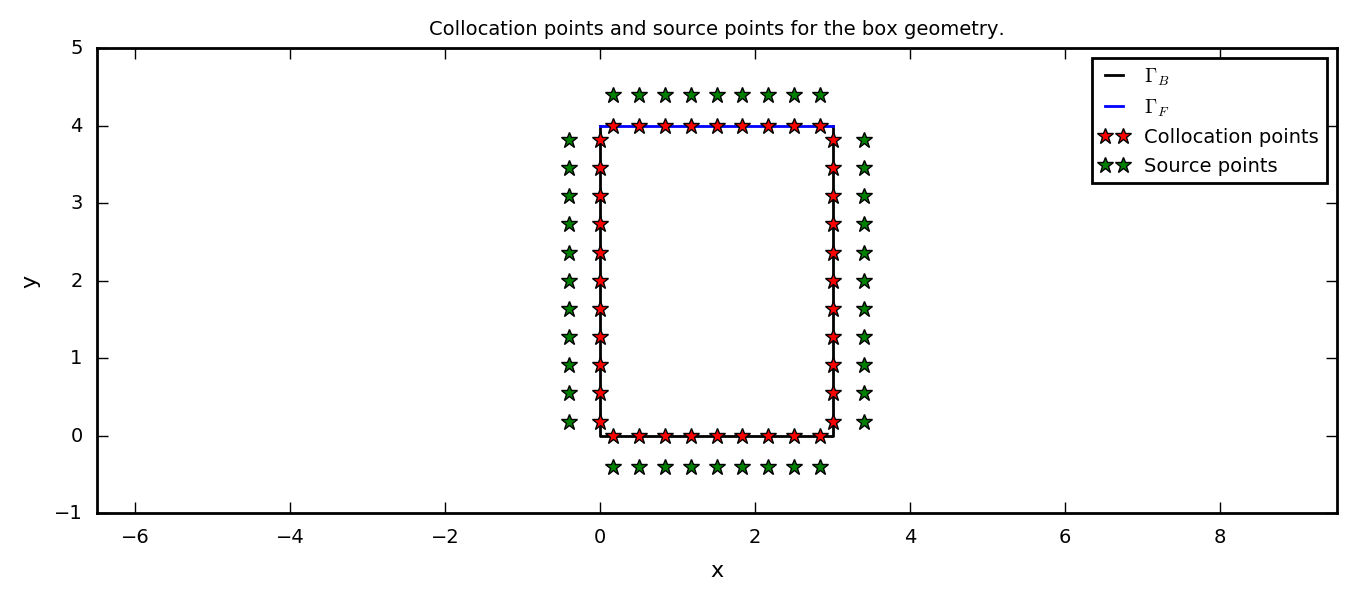### 4.1 Circular geometry.¶

Our circular geometry is going to be parameterized by \begin{align} \boldsymbol C_\text B &= \boldsymbol e_x[x_0+R\sin(\xi+\theta/2)] +\boldsymbol e_y[y_0+R\cos(\xi+\theta/2)], \quad 0\leq \xi\leq 2\pi - \theta,\\ \boldsymbol C_\text F &= \boldsymbol e_x[x_0+R\sin(\theta/2)-xi] +\boldsymbol e_y[y_0+R\cos(\xi+\theta/2)], \quad 0< \xi< 2R\sin(\theta/2). \end{align} The collocation points and the source points can be calculated by the following function.

In :
def csPointsCircle(N,x0,y0,theta,delta):
"""
N:      int. Number of source and collocation points.
delta:  float. Distance between the source and collocation points.
Returns:
xc:     float arr. 2xN array of the collocation points on the container.
xs:     float arr. 2xN array of the corresponding source points.
n:      float arr. The unit normal vectors at the collocation points.
"""
# Number of source and collocation points on the container (NB) and the surface (NF).
circumference = R*(2*np.pi-theta+2*np.sin(theta/2))
NF = round(N*R*2*np.sin(theta/2)/circumference)
NB = N-NF

# Angular spacing between the collocation points on the container.
dtheta = (2*np.pi-theta)/NB
# Spacing between the collocation points on the surface.
dF = 2*R*np.sin(theta/2)/NF

# Calculates the collocation points, and saves them in a 2xN matrix.
xc = np.zeros((2,N))
# NB
xi1 = np.arange(dtheta/2,2*np.pi-theta,dtheta)
xc[0,0:NB] = x0 + R*np.sin(xi1 + theta/2)
xc[1,0:NB] = y0 + R*np.cos(xi1 + theta/2)
# NF
xi2 = np.arange(dF/2,2*R*np.sin(theta/2),dF)
xc[0,NB:N] = x0 + R*np.sin(theta/2) - xi2
xc[1,NB:N] = y0 + R*np.cos(theta/2)

# The unit normal vector of the container.
n = np.zeros((2,N))
n[0,0:NB] = np.sin(xi1 + theta/2)
n[1,0:NB] = np.cos(xi1 + theta/2)
n[1,NB:N] = 1

# Calculates the source points, and saves them in a 2xN matrix.
xs = xc + delta*n

return xc, xs, n


We visualize the result as we did for the box geometry.

In :
R = 2 # Radius.
theta = np.pi/2 # Opening angle
x0, y0 = 2, 2 # Center of the circle.
N = 40 # Number of collocation and source points.
delta = 0.4 # Distance between the source and collocation points.

# Calculates the collocation points and the source points.
xc, xs, n = csPointsCircle(N,x0,y0,theta,delta)

# Visualize the results.
# Plotting the container and the fluid surface.
xi = np.linspace(0,2*np.pi-theta,100,endpoint=True)
plt.plot(x0 + R*np.sin(xi + theta/2), y0 + R*np.cos(xi + theta/2),'k',label=r'$\Gamma_B$'); # The container.
plt.plot([x0 + R*np.sin(theta/2), x0 - R*np.sin(theta/2)],
[y0 + R*np.cos(theta/2), y0 + R*np.cos(theta/2)],'b',label=r'$\Gamma_F$'); # The fluid surface.
# Plotting collocation and source points.
plt.plot(xc,xc,'r*',label='Collocation points')
plt.plot(xs,xs,'g*',label='Source points')
# Figure texts.
plt.title('Collocation points and source points for the circular geometry.')
plt.xlabel('x'), plt.ylabel('y'), plt.axis('equal'), plt.legend();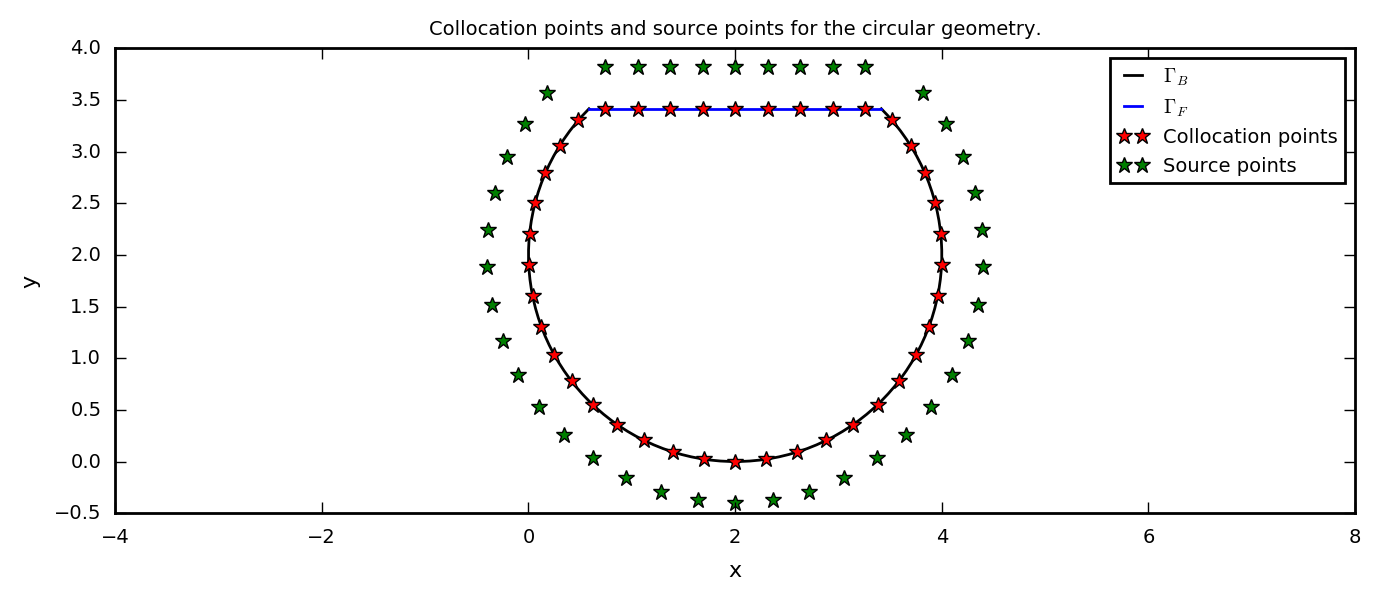### 4.3 General geometry.¶

We are going to define a general geometry through polynomial curve interpolation of a set of data points. Polynomial interpolation is explained in the module Polynomial Interpolation at numfys. We are going to use Lagrange interpolation.

We start of by defining a function that performs the interpolation.

In :
def curveInterpolation(x_data,y_data,N):
""" Interpolates a curve through the points (x_data,y_data) and returns a
curve C(xi), where 0<xi<1.
Parameters:
x_data: float arr. All the x-values of the data set.
y_data: float arr. All the y-values of the data set.
x_data and y_data has to be of the same length.
N:      float arr. Number of parameter values.
Returns:
[Cx,Cy]:float arr. The interpolation curve.
"""
n = min(len(x_data),len(y_data))
t = np.linspace(0,1,N,endpoint=True) # Parameter.

# Chebychev nodes.
a = (np.cos(np.pi/(2*n))-1)/(2*np.cos(np.pi/(2*n)))
b = (np.cos(np.pi/(2*n))+1)/(2*np.cos(np.pi/(2*n)))
t_i = (b + a)/2 + (b - a)/2*np.cos((2*n-2*np.linspace(1,n,n)+1)*np.pi/(2*n))

# Performing the interpolation.
L = *n
for i in range(0,n):
for j in range(0,n):
if (j!=i):
L[j]=L[j]*(t - t_i[i])/(t_i[j] - t_i[i])
Cx, Cy = t*0, t*0
for i in range(0,n):
Cx = Cx + x_data[i]*L[i]
Cy = Cy + y_data[i]*L[i]

return [Cx,Cy]


As before, we need to find the unit normal vectors so that the source points may be calculated. Thus, we need to calculate the derivative of the interpolation curve. This can for example be achieved by derivating the Lagrange interpolation formula (see the module on polynomial interpolation), which yields $$\boldsymbol C'_\text B (t) = \sum_{j=1}^n\boldsymbol P_j L'_j(t),$$ $$L_j'(t)=\sum_{i=1,i\neq j}^n\frac{1}{t_j-t_i}\prod_{k=1, k\neq i,j}^n\frac{t-t_k}{t_j-t_k}.$$ Using the fact that $\boldsymbol n\cdot \boldsymbol C'=0\wedge ||\boldsymbol n||= 1$, one can easily calculate the normal unit vectors $\boldsymbol n(t)=[n_x(t),n_y(t)]$. Let us create a function that calculates the unit normal vectors and the derivative of the interpolation polynomial.

In :
def diffCurveInterpolation(x_data,y_data,N):
""" Calculates the derivative of a curve that tnterpolates the points
(x_data,y_data) dC(t) and the unit normal vectors n(t).
Parameters:
x_data:    float arr. All the x-values of the data set.
y_data:    float arr. All the y-values of the data set.
x_data and y_data has to be of the same length.
N:         float arr. Number of parameter values.
Returns:
[dCx,dCy]: float arr. The derivative of the interpolation curve.
[nx,ny]:   float arr. The unit normal vectors.
"""
n = min(len(x_data),len(y_data))
t = np.linspace(0,1,N,endpoint=True) # Parameter.

# Chebychev nodes.
a = (np.cos(np.pi/(2*n))-1)/(2*np.cos(np.pi/(2*n)))
b = (np.cos(np.pi/(2*n))+1)/(2*np.cos(np.pi/(2*n)))
t_i = (b + a)/2 + (b - a)/2*np.cos((2*n-2*np.linspace(1,n,n)+1)*np.pi/(2*n))

# Calculating the derivative of the interpolation.
dL = *n
for i in range(0,n):
for j in range(0,n):
if (j!=i):
multi = np.ones(len(t))
for k in range(0,n):
if (j!=k and i!=k and j!=i):
multi = multi*(t - t_i[k])/(t_i[j] - t_i[k])
dL[j] = dL[j] + 1/(t_i[j] - t_i[i])*multi
dCx, dCy = t*0, t*0
for i in range(0,n):
dCy = dCy + y_data[i]*dL[i]
dCx = dCx + x_data[i]*dL[i]

# Calculating the unit normal vectors.
nx = dCy/np.sqrt(dCx**2+dCy**2)
ny = -dCx/np.sqrt(dCx**2+dCy**2)

return [dCx,dCy], [nx,ny]


We also need to perform some numerical integrations, which we will use to calculate the length of the container and to uniformly distribute the collocation points. There are many packages for python which includes such functions (e.g. scipy.integrate.simps()), but we are going to create our own. More specifically, we are going to use Simpsons method (one step) and the composite Simpsons method (many steps). Check out the modules on numerical integration at numfys for more detailed information about Simpsons method.

In :
def compositeSimpsonsMethod(y,a,b):
""" Uses the composite Simpsons method to calculate the definite integral of y(x). The
input y is the y-values at some x-values, which are assumed to be uniformly distributed
between (and included) a and b.
Parameters:
y:    Float arr. y-values of the function evaluated at uniformly distributed x-values.
a:    Float. Start value for integration.
b:    Float. End value for integration.
Returns:
I:    Float. Approximation of the definite integral.
"""
n = len(y)-1 # Number of sub-intervals
h = (b-a)/n # The assumed distance between the function evaluations y(x).
# Performing the composite simpsons method.
odd, even = 0, 0
for i in range(1,n,2):
odd += y[i]
for i in range(2,n,2):
even += y[i]
I = h/3*(y + y[n] + 4*odd + 2*even)
return I

def simpleSimpsonsMethod(y0,y1,y2,dx):
""" Uses the Simpsons method to calculate the definite integral of y(x) using the three
function evaluations y0, y1, y2 at some x-values with a distance dx.
Parameters:
y0: Float. First y-value.
y1: Float. Second y-value.
y2: Float. Third y-value.
dx: Float. Assumed destance between the function evaluations.
Returns:
Float. Approximation of the definite integral.
"""
return dx/3*(y0+4*y1+y2)


Now we have the tools to define a container from a set of data points, distribute the collocation points uniformly and then calculate the source points.

For simplicity, let us assume that the first and last data point is on the same horizontal level. Then, $\Gamma_\text F$ is simply defined as the line between these two points. The collocation points on $\Gamma_\text F$ and the corresponding source points are then calculated as previously.

The $N$ collocation points are distributed bewteen $\Gamma_\text F$ and $\Gamma_\text B$ such that the collocation points are distributed uniformly. The length of $\Gamma_\text B$ is found by integrating the derivative of the curve, $$L_B = \int_{t_0}^{t_1} ||\boldsymbol C'_\text B(t)|| \,\text dt.$$

The collocation points of $\Gamma_\text B$ is distributed with the following algorithm: Let $L_\text B$ be the length of $\Gamma_\text B$. Then, the parameter value between each collocation point is given by $L_\text B/N_\text B$, which in turn can be calculated by $$\int_{\hat{t}_{i-1}}^{\hat{t}_i} ||\boldsymbol C'_\text B(t)|| \,\text dt = \frac{L_\text B}{N_\text B}.$$ The goal is to calculate $\hat t_i$, which is the parameter value at which the $i$th collocation point is to be placed. This can for example be achieved by performing Simpsons method between three and three parameter values and then checking if the integral is correct. The first collocation point is to be placed a distance $L_\text B/2 N_\text B$ from the beginnning.

With all this settled, we are finally ready to create a function that calculates the collocation points and source points of the general geometry.

In :
def csPointsGeneral(x_data,y_data,N,delta,Nt):
""" Calculates source and collocation points of the general geometry. The general geometry
is defined as the polynomial interpolation of (x_data,y_data). The first and the last element
of y_data is assumed to be equal, such that a horizontal line between the first and the last
data point can be achieved.
Parameters:
x_data: float arr. All the x-values of the data set.
y_data: float arr. All the y-values of the data set.
x_data and y_data has to be of the same length.
N:      int. Number of collocation and source points.
delta:  float. Distance between the source and collocation points.
Nt:     float. Number of parameter increments of the curve describing the general geometry.
Returns:
xc:     float arr. 2xN array of the collocation points on the container.
xs:     float arr. 2xN array of the corresponding source points.
[nx,ny]:float arr. The unit normal vectors at the collocation points.
NB:     int. Number of collocation points on the container.

"""
n = min(len(x_data),len(y_data))

t = np.linspace(0,1,Nt,endpoint=True) # Parameter.
dt = 1/Nt # Parameter increment.

# The curve describing the container.
[CBx,CBy] = curveInterpolation(x_data,y_data,Nt)
# The derivative of the container and its unit normal vectors.
[dCxB,dCyB], [nxB,nyB] = diffCurveInterpolation(x_data,y_data,Nt)

# Length of the container.
vel = np.sqrt(dCyB**2+dCxB**2)
LB = compositeSimpsonsMethod(vel,0,1)
# Length of the fluid surface.
LF = abs(x_data-x_data[n-1])

# Distribution of collocation points.
NB = int(N*LB/(LB+LF))
NF = N-NB

# Using Simpsons method to distibute the collocation points on the container as described
# in the text.
tHat = *NB # The parameter indices at which the collocation points are to be placed.
count = 0
# First collocation point.
I = 0
while (I<=LB/(2*NB)):
I += simpleSimpsonsMethod(vel[count],vel[count + 1],vel[count + 2],dt)
count += 2
tHat = count - 1
# The rest of the collocation points.
for j in range(1,NB):
I = 0
while (I<=LB/(NB) and count < Nt-2):
I += simpleSimpsonsMethod(vel[count],vel[count + 1],vel[count + 2],dt)
count += 2
tHat[j] = count - 1

# The collocation points.
xc = np.zeros((2,N))
# On the container.
xc[0,0:NB], xc[1,0:NB] = CBx[tHat], CBy[tHat]
# On the fluid surface.
xc[0,NB:N], xc[1,NB:N] = x_data + np.arange(LF/(2*NF),LF,LF/NF), y_data

# The unit normal vectors.
n = np.zeros((2,N))
# On the container.
n[0,0:NB], n[1,0:NB] = nxB[tHat], nyB[tHat]
# On the fluid surface.
n[0,NB:N], n[1,NB:N] = 0, 1

# The source points.
xs = n*delta + xc

return xc, xs, n, NB


It is finally time to visualize the source and collocation points for the general geometry, and not least the geometry itself.

In :
# Define some data points.
x_data = [1.5,0.8,0.8,1.2,1.5,2.5,3.6,3.8,4.2,4.1,3.8]
y_data = [4.5,3.7,2.5,1.5,0.9,0.4,1.0,2.0,3.1,4.0,4.5]
n = len(x_data) # Number of data points.
N = 40 # Number of collocation and source points.
delta = 0.4 # Distance between the source and collocation points.
Nt = N**3 # Number of parameter increments of the curve describing the general geometry.

# Calculating the source and collocation points.
xc, xs, dummy, dummy = csPointsGeneral(x_data,y_data,N,delta,Nt)

# Visualize the results.
# Plotting the container and the fluid surface.
[Cx,Cy] = curveInterpolation(x_data,y_data,100)
plt.plot(Cx,Cy,'k',label=r'$\Gamma_B$')
plt.plot([x_data,x_data[n-1]],[y_data,y_data[n-1]],'b',label=r'$\Gamma_B$')
# Plotting collocation and source points.
plt.plot(xc,xc,'r*',label='Collocation points')
plt.plot(xs,xs,'g*',label='Source points')
# Figure texts.
plt.title('Collocation points and source points for a general geometry.')
plt.xlabel('x'), plt.ylabel('y'), plt.axis('equal'), plt.legend();

# Plot the data points and set figure texts.
plt.plot(x_data,y_data,'m.',label='Data points.')
plt.title('Polynomial curve interpolation of a set data points.')
plt.legend(), plt.xlabel('x'), plt.ylabel('y');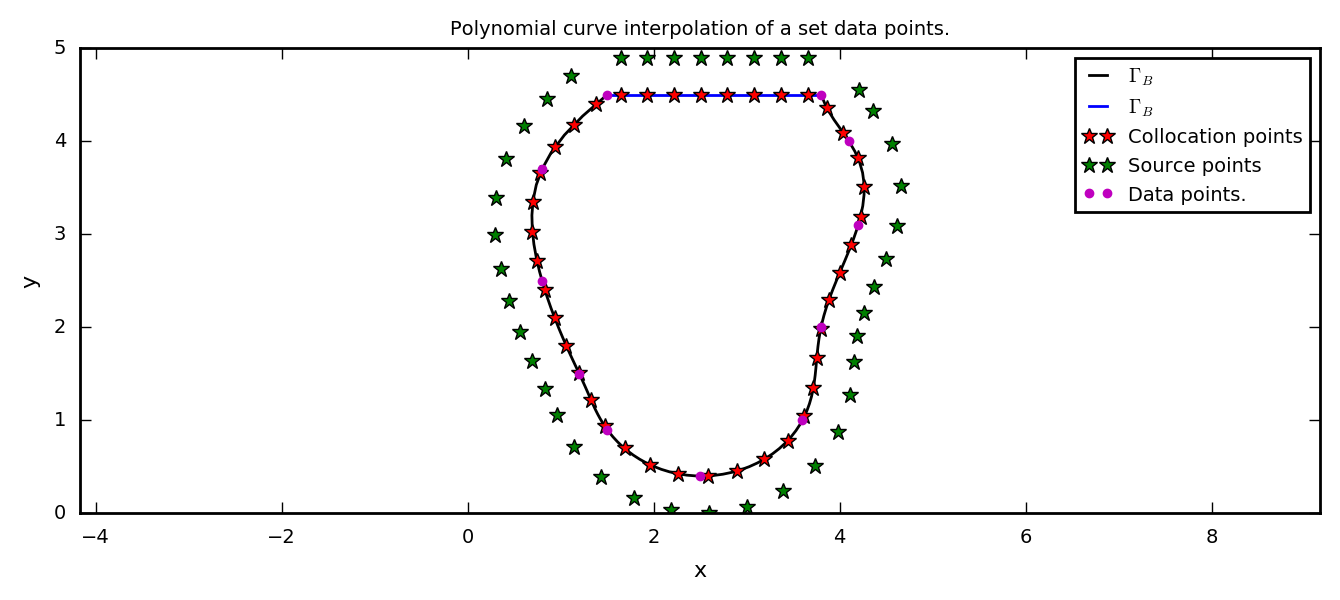## 5 Solving the mixed Steklov eigenvalue problem and plotting the waves.¶

Now, everything is set to solve the mixed Steklov eigenvalue problem for our setup. As mentioned earlier, we are going to use the function scipy.linalg.eig() to solve the eigenvalue problem $A\boldsymbol \alpha = \lambda B \boldsymbol \alpha$ aquired previously. Note at $B$ is singular (which implies that $B\boldsymbol{\alpha} = 0$ has non-trivial solutions), but $A-\lambda B \approx -\lambda B$ for large $|\lambda|$. As a consequence, scipy.linalg.eig() may produce the eigenvalues $\pm \infty$. We are going to dismiss these eigenvalues, and sort the rest (finite) values, such that we easily can analyze the waves corresponding to the lowest eigenvalues.

### 5.1 Box geometry. Analytical solution.¶

To start with, let us find the collocation points, the source points and the matrices $A$ and $B$ as described earlier. $N$ and $\delta$ are chosen after some trial and error.

In :
L = 3.0 # Length of the box.
H = 4.0 # Height of the box.
N = 100 # Number of collocation and source points.
delta = 0.5 # Distance between the source and collocation points.

# Calculates the collocation points and the source points.
xc, xs = csPointsBox(N,L,H,delta)

# Number of source and collocation points on the different sides. N=2*NL+2*NH.
NH = int(round(N*H/(2*L + 2*H))) # On the vertical lines.
NL = int(N/2 - NH) # On the horizontal lines.

# The unit normal vector of the container in the collocation points.
n = np.zeros((2,N))
n[0,0:NH] = -np.ones(NH)
n[0,NH + NL:2*NH + NL] = np.ones(NH)
n[1,NH:NH + NL] = -np.ones(NL)
n[1,2*NH + NL:N] = np.ones(NL)

# The A and B matrices.
A = np.zeros((N,N))
B = np.zeros((N,N))
for i in range(0,N):
A[i,:] = ((n[0,i]*(xc[0,i] - xs[0,:]) + n[1,i]*(xc[1,i]-xs[1,:]))/
((xc[0,i] - xs[0,:])**2 + (xc[1,i] - xs[1,:])**2))
for i in range(2*NH + NL,2*NH + 2*NL):
B[i,:] = np.log(np.sqrt((xc[0,i] - xs[0,:])**2 + (xc[1,i] - xs[1,:])**2))


scipy.linalg.eig() solves an ordinary or generalized eigenvalue problem of a square matrix. In our case, we want to solve $A\boldsymbol \alpha = \lambda B \boldsymbol \alpha$, and has to call the function as

In :
# Solve the eigenvalue problem.
lamb,alph = la.eig(A,B,left=0,right=1)


We now want to ignore $\lambda\leq0$ and $\lambda = \pm \infty$, and then sort the eigenvalues and the corresponding eigenvectors. We create a function that does this, so that we don't repeate ourselves.

In :
def validLambdaAlpha(lamb,alph):
""" Seperates out unvalid eigenvalues and returns a sorted list of valid eigenvalues.
Parameters:
lamb:       float arr. List of eigenvalues.
alph:       float arr. Array of eigenvectors corresponding to the eigevalues.
Each row holds a eigevector.
Returns:
sortLamb:   float arr. sorted list of valid eigenvalues.
sortAlph:   float arr. Array of sorted eigenvectors corresponding  to the eigevalues.
Each row holds a eigevector.
"""
# Check for valid eigenvalues, and save them and the corresponding eigenvectors in two arrays.
# All of our eigenvalues should be real, but the eig-function returns
# returns imaginary numbers (a+0j) for the eigenvalues.
validAlph = [] # Valid eigenvectors and eigenvalues.
validLamb = []
count = 0 # Number of valid eigenvectors and eigenvalues.
for i in range(0,N):
if lamb[i] > 0 and np.isfinite(lamb[i]):
validLamb.append(float(lamb[i]))
validAlph.append(alph[:,i])
count+=1

# Sort the eigenvalues and eigenvector on the value of the eigenvalues.
sortIndex = np.argsort(validLamb)
sortLamb = validLamb.copy()
sortAlph = validAlph.copy()
for i in range(0,count):
sortLamb[i] = validLamb[sortIndex[i]]
sortAlph[i] = validAlph[sortIndex[i]]
return sortLamb, sortAlph


For this box geometry the analytical solution is known. It is $$\psi_n(x,y)=A_n\cos\frac{n\pi x}{L}\cosh\frac{n\pi y}{L},$$ with corresponding eigenvalues $$\lambda_n = \frac{n\pi}{L}\tanh\frac{n\pi H}{L}.$$ This is easily shown using for example seperation of variables. Note that $A_n$ are unknown parameters (amplitudes), but we are going to normalize the waves such that they get an amplitude of 1. Since we know the analytical solution, we are going to compare the numerical solution and the analytical solution.

In :
# The sorted numerical eigenvalues and eigenvectors.
validLamb, validAlph = validLambdaAlpha(lamb,alph)
# The analytical eigenvalues.
analyticalLamb = np.arange(1,len(validLamb) + 1)*np.pi/L*np.tanh(
np.arange(1,len(validLamb) + 1)*np.pi*H/L)
# The relative error between the analytical and the numerical eigenvalues.
relError = abs((analyticalLamb - validLamb)/analyticalLamb)
# Printing the results as a HTML table.
table = ["<table>",
"<td><b>Node</b></td>",
"<td><b>Numerical eigenvalue</b></td>",
"<td><b>Analytitcal eigenvalue</b></td>",
"<td><b>Relative error</b></td>"]
for i in range(0,min(len(validLamb),10)):
table.append("<tr><td>")
table.append(str(i + 1))
table.append("</td><td>")
table.append("%.10f" % float(validLamb[i]))
table.append("</td><td>")
table.append("%.10f" % float(analyticalLamb[i]))
table.append("</td><td>")
table.append("%.5f %%" % (float(relError[i])*100))
table.append("</td></tr>")
table.append("</table>")
HTML(''.join(table))

Out:
 Node Numerical eigenvalue Analytitcal eigenvalue Relative error 1 1.0473213837 1.0467160229 0.05783 % 2 2.0950956350 2.0943948809 0.03346 % 3 3.1420004846 3.1415926535 0.01298 % 4 4.1875681223 4.1887902048 0.02918 % 5 5.2316574583 5.2359877560 0.08270 % 6 6.2727832704 6.2831853072 0.16555 % 7 7.3104286633 7.3303828584 0.27221 % 8 8.3421946077 8.3775804096 0.42239 % 9 9.3669984472 9.4247779608 0.61306 % 10 10.3811366757 10.4719755120 0.86745 %

Let us plot the waves!

In :
x = np.linspace(0,L,100,endpoint=True) # x-axis.
psi = np.zeros(len(x)) # Allocate the wave function, psi.

for node in [1,2,3,4]:
# Calculate the wave fucntion using.
psi = np.zeros(len(x))
for n in range(0,N):
psi = psi + validAlph[node - 1][n]*np.log(np.sqrt(
(x - xs[0,n])**2 + (H - xs[1,n])**2 ));
# Calculate h and normalize such that the amplitude becomes 1.
h = psi/np.max(psi)*np.sign(psi)

# Calculate the theoretical curve.
psiT = np.cos(node*np.pi*x/L)*np.cosh(node*np.pi*H/L)
hT = psiT/np.max(psiT)*np.sign(psiT)

# Calculate the maximal absolute error of the normalized h functions.
absError = np.max(np.abs(h-hT))

# Plot the result.
plt.figure()
plt.plot(x,hT+H,'r',label='Analytical solution.')
plt.plot(x,h+H,'b',label='Numerical solution.')
plt.xlabel('x'); plt.ylabel('y');
plt.title('%d. node.' % node) ,plt.legend();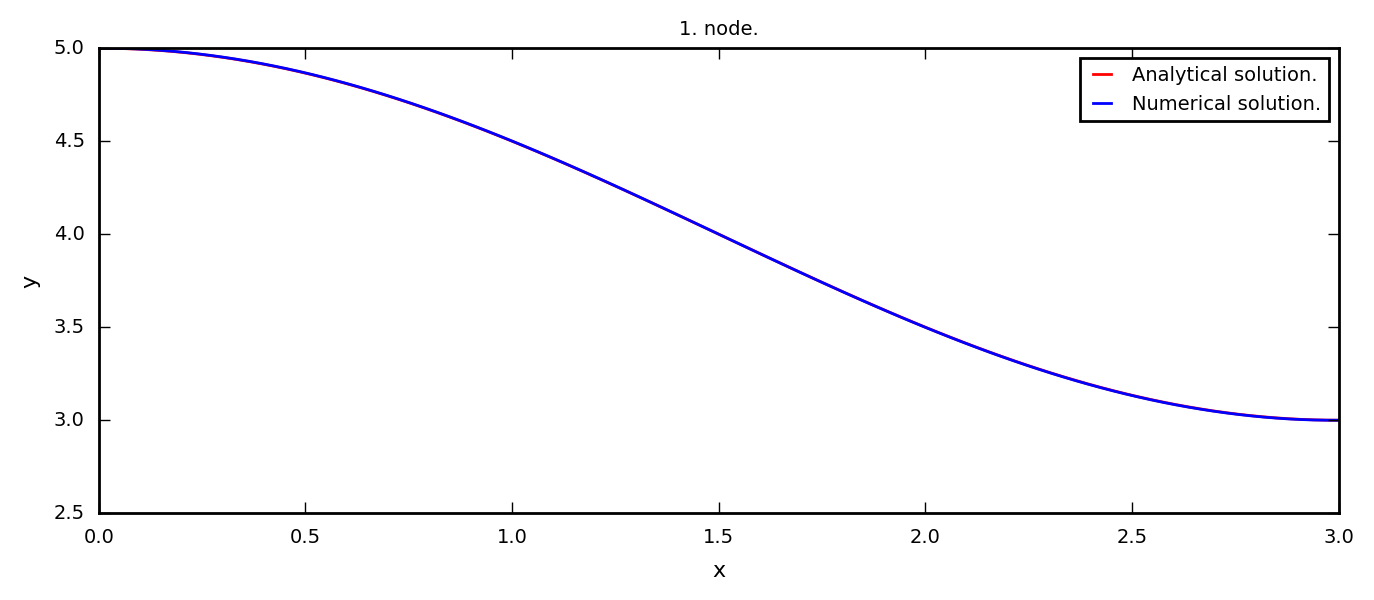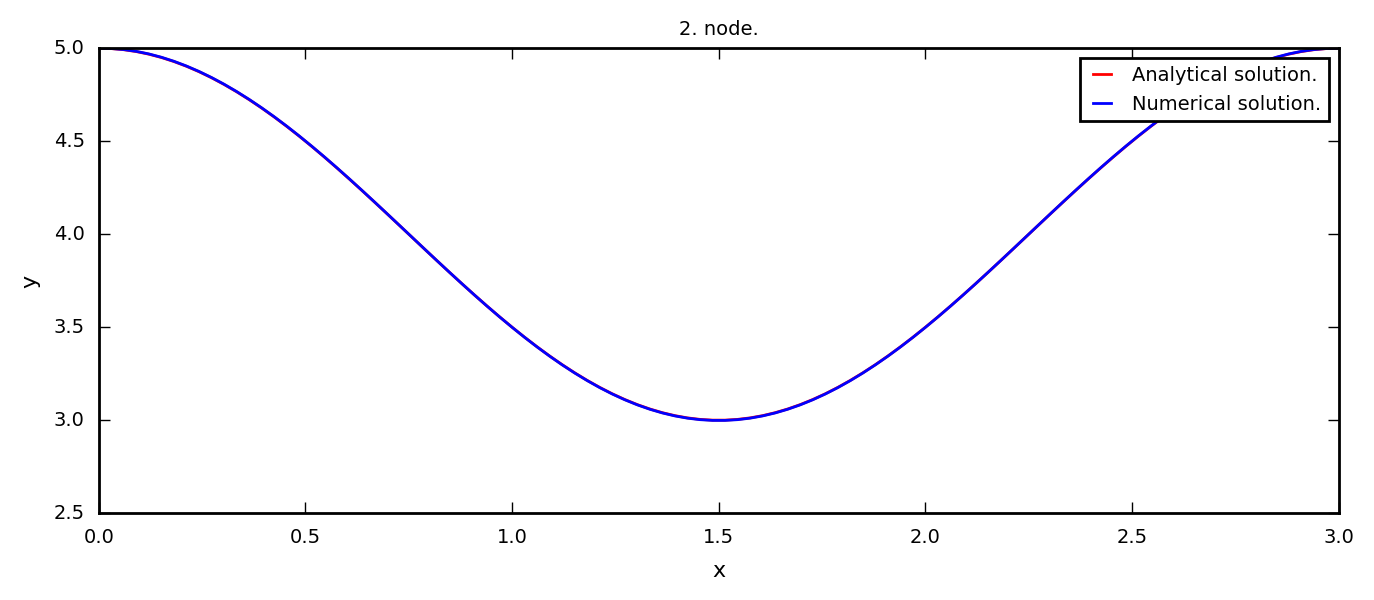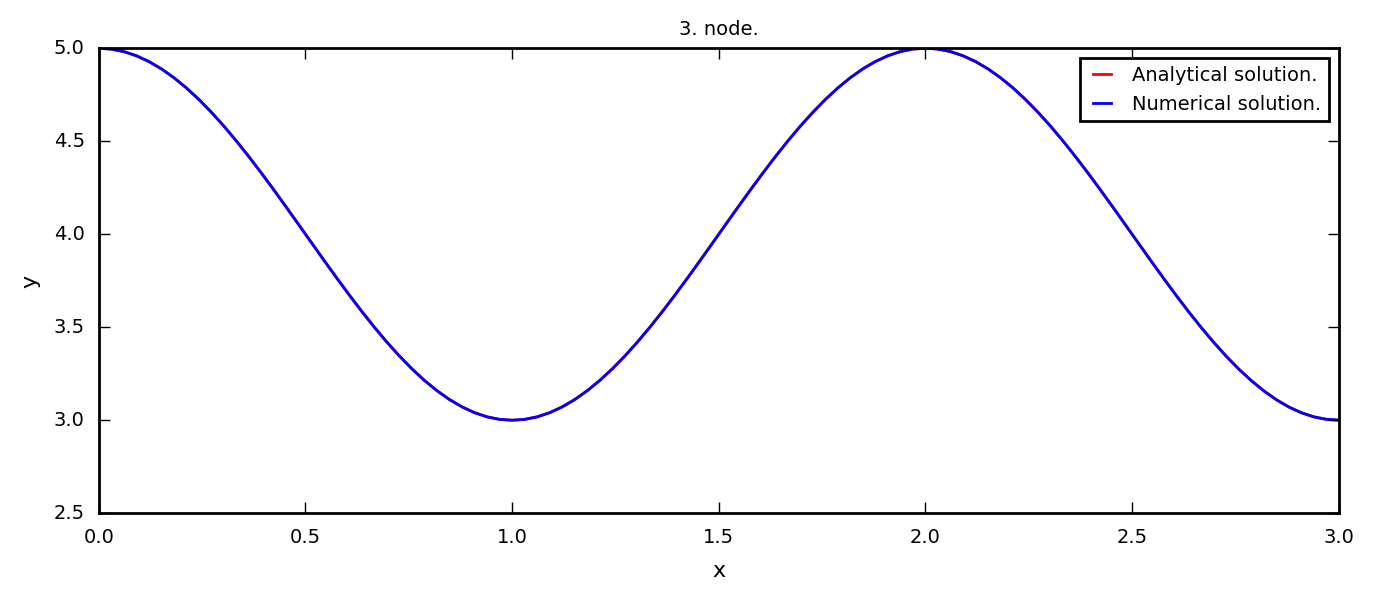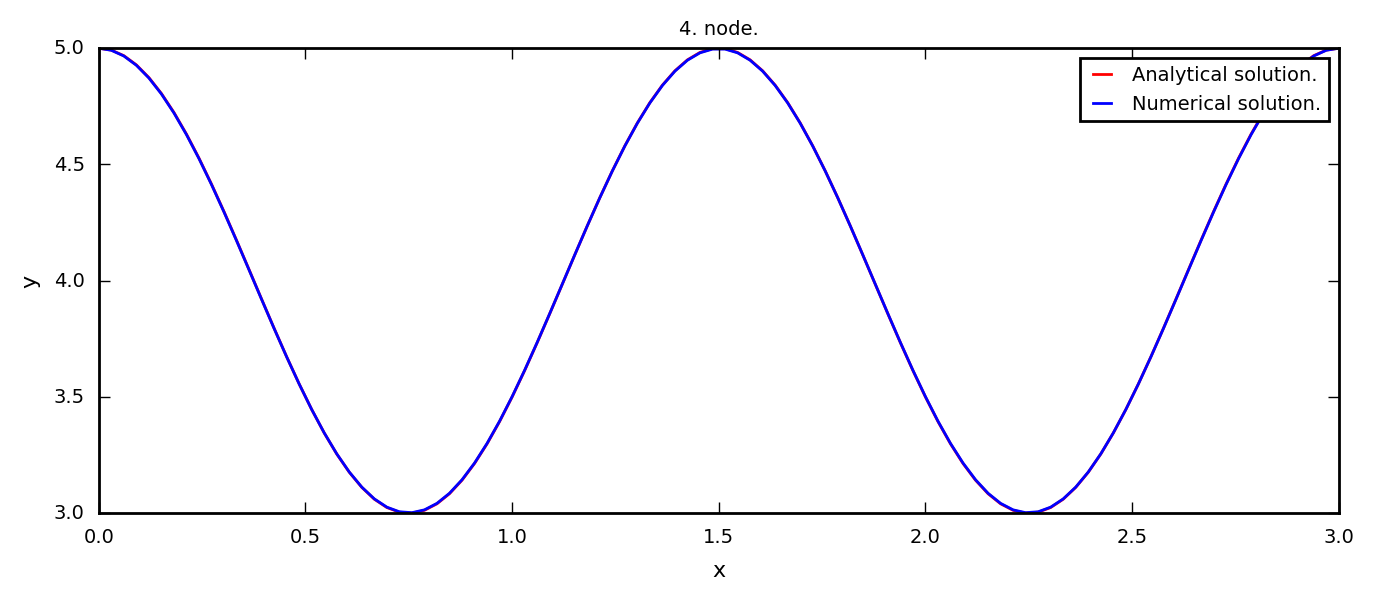### 5.2 Circular geometry.¶

We now repeate the process for the cirvular geometry.

In :
R = 2 # Radius.
theta = np.pi/2 # Opening angle
x0, y0 = 2, 2 # Center of the circle.
N = 100 # Number of collocation and source points.
delta = 0.5 # Distance between the source and collocation points.
H = y0 + R*np.cos(theta/2)
# Number of source and collocation points on the container (NB) and the surface (NF).
circumference = R*(2*np.pi-theta+2*np.sin(theta/2))
NF = int(round(N*R*2*np.sin(theta/2)/circumference))
NB = N-NF

# Calculates the collocation points and the source points.
xc, xs, n = csPointsCircle(N,x0,y0,theta,delta)

# The A and B matrices.
A = np.zeros((N,N))
B = np.zeros((N,N))
for i in range(0,N):
A[i,:] = ((n[0,i]*(xc[0,i] - xs[0,:]) + n[1,i]*(xc[1,i]-xs[1,:]))/
((xc[0,i] - xs[0,:])**2 + (xc[1,i] - xs[1,:])**2))
for i in range(NB,N):
B[i,:] = np.log(np.sqrt((xc[0,i] - xs[0,:])**2 + (xc[1,i] - xs[1,:])**2))

# Solve the eigenvalue problem.
lamb,alph = la.eig(A,B,left=0,right=1)

# The sorted numerical eigenvalues and eigenvectors.
validLamb, validAlph = validLambdaAlpha(lamb,alph)
# The analytical eigenvalues.
analyticalLamb = np.arange(1,len(validLamb) + 1)*np.pi/L*np.tanh(
np.arange(1,len(validLamb) + 1)*np.pi*H/L)
# The relative error between the analytical and the numerical eigenvalues.
relError = abs((analyticalLamb - validLamb)/analyticalLamb)
# Printing the results as a HTML table.
table = ["<table>",
"<td><b>Node</b></td>",
"<td><b>Numerical eigenvalue</b></td>"]
for i in range(0,min(len(validLamb),10)):
table.append("<tr><td>")
table.append(str(i + 1))
table.append("</td><td>")
table.append("%.10f" % float(validLamb[i]))
table.append("</td></tr>")
table.append("</table>")
HTML(''.join(table))

Out:
 Node Numerical eigenvalue 1 1.2422903215 2 2.3644793944 3 3.4944615692 4 4.5955198621 5 5.7104584323 6 6.8086237056 7 7.9153817971 8 9.0097226355 9 10.1085608825 10 11.1963750286
In :
x = np.linspace(x0 - R*np.sin(theta/2), x0 + R*np.sin(theta/2),100,endpoint=True) # x-axis.
psi = np.zeros(len(x)) # Allocate the wave function, psi.

for node in [1,2,3,4]:
# Calculate the wave fucntion using.
psi = np.zeros(len(x))
for n in range(0,N):
psi = psi + validAlph[node - 1][n]*np.log(np.sqrt(
(x - xs[0,n])**2 + (H - xs[1,n])**2 ));
# Calculate h and normalize such that the amplitude becomes 1.
h = psi/np.max(psi)*np.sign(psi)

# Plot the result.
plt.figure()
plt.plot(x,h+H,'b',label='Numerical solution.')
plt.xlabel('x'); plt.ylabel('y');
plt.title('%d. node.' % node), plt.legend();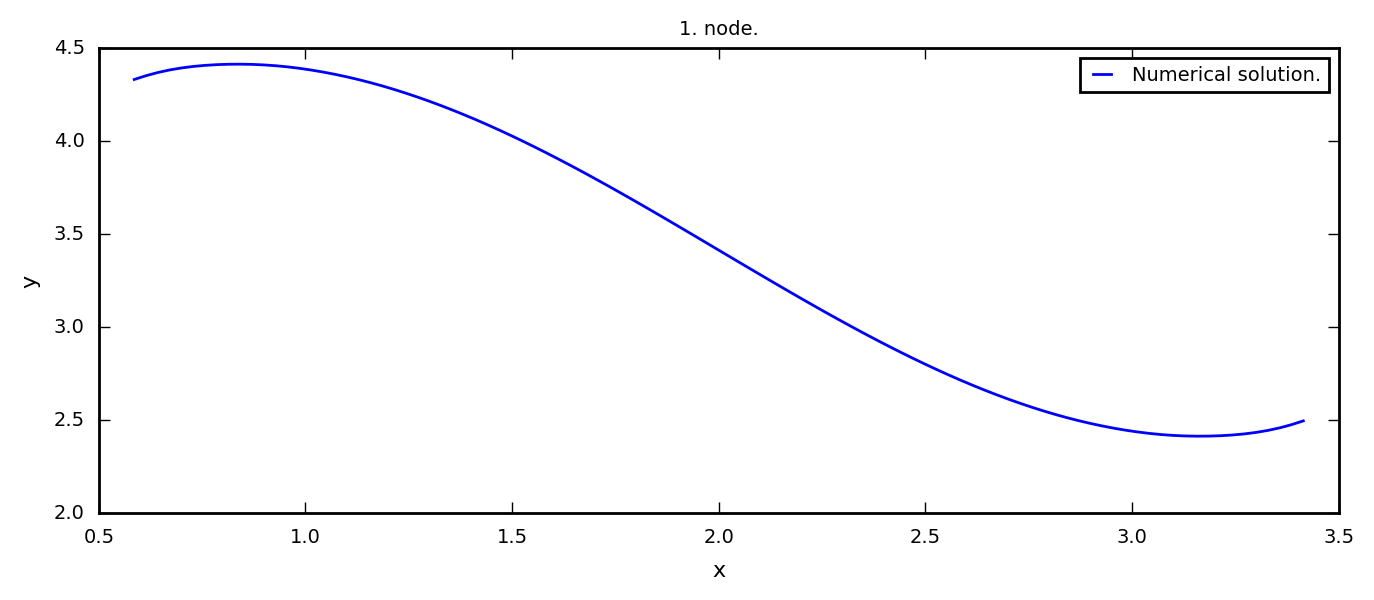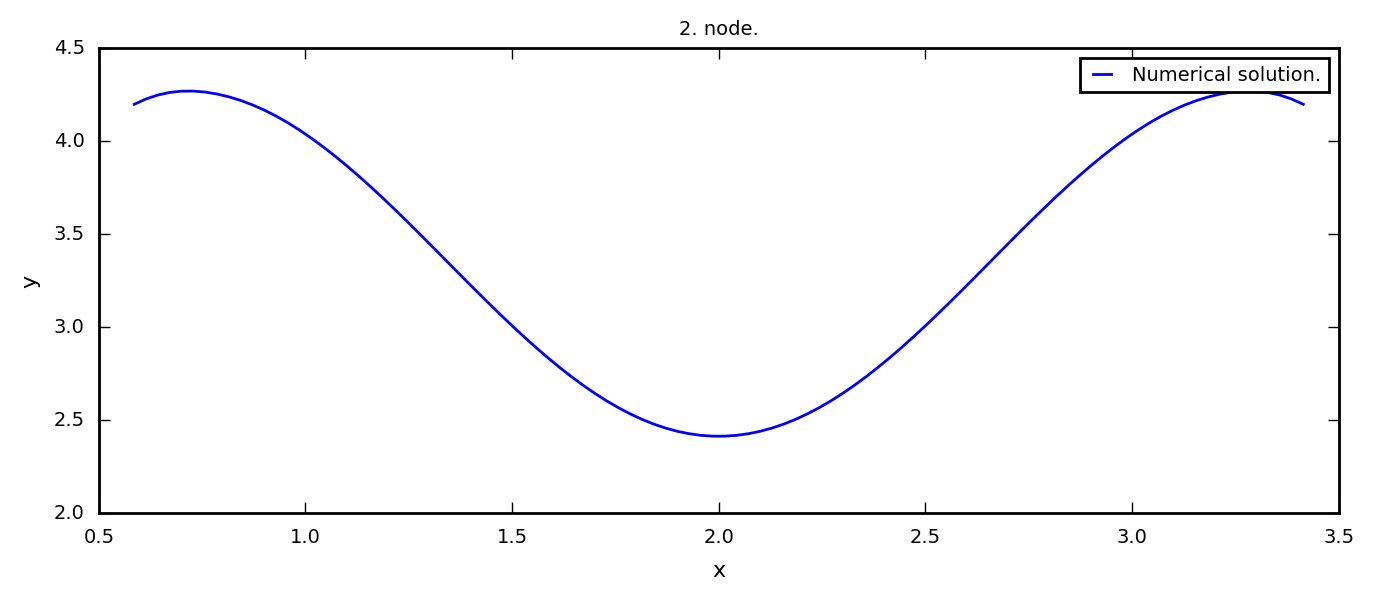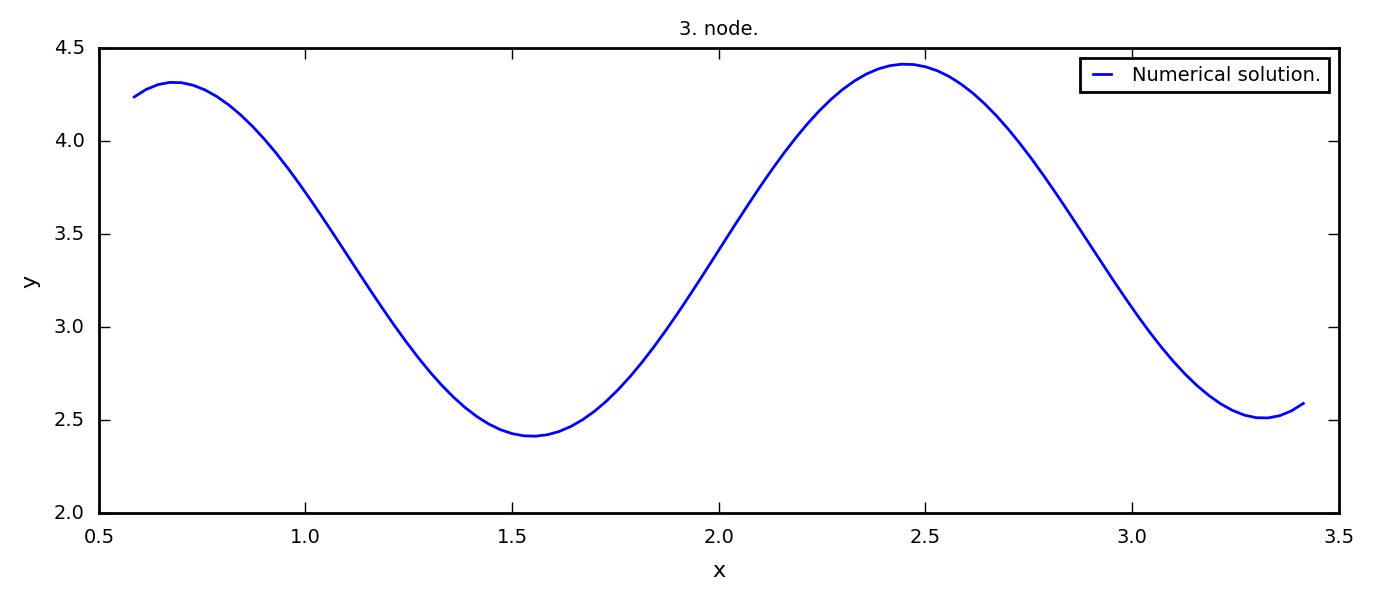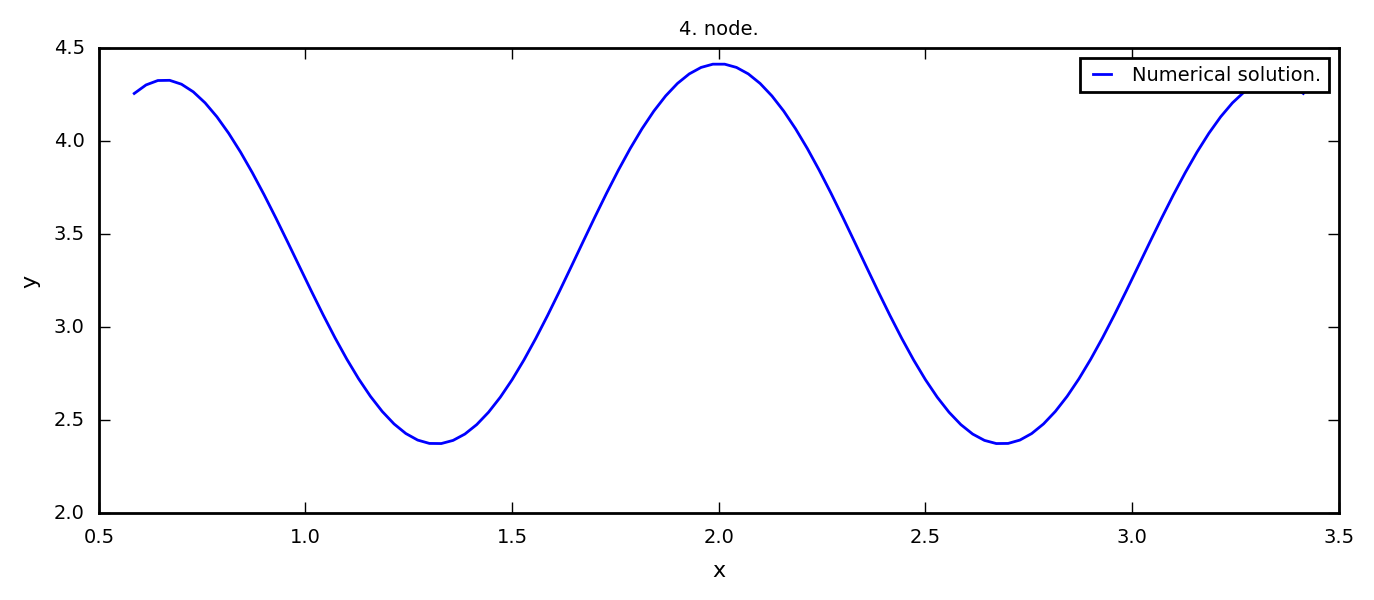### 5.3 General geometry.¶

We now repeate the process for the general geometry.

In :
# Define some data points.
x_data = [1.5,0.8,0.8,1.2,1.5,2.5,3.6,3.8,4.2,4.1,3.8]
y_data = [4.5,3.7,2.5,1.5,0.9,0.4,1.0,2.0,3.1,4.0,4.5]
n = len(x_data) # Number of data points.
N = 100 # Number of collocation and source points.
delta = 0.4 # Distance between the source and collocation points.
Nt = N**2 # Number of parameter increments of the curve.
H = y_data

# Calculating the source and collocation points.
xc, xs, n, NB = csPointsGeneral(x_data,y_data,N,delta,Nt)

# The A and B matrices.
A = np.zeros((N,N))
B = np.zeros((N,N))
for i in range(0,N):
A[i,:] = ((n[0,i]*(xc[0,i] - xs[0,:]) + n[1,i]*(xc[1,i]-xs[1,:]))/
((xc[0,i] - xs[0,:])**2 + (xc[1,i] - xs[1,:])**2))
for i in range(NB,N):
B[i,:] = np.log(np.sqrt((xc[0,i] - xs[0,:])**2 + (xc[1,i] - xs[1,:])**2))

# Solve the eigenvalue problem.
lamb,alph = la.eig(A,B,left=0,right=1)

# The sorted numerical eigenvalues and eigenvectors.
validLamb, validAlph = validLambdaAlpha(lamb,alph)
# The analytical eigenvalues.
analyticalLamb = np.arange(1,len(validLamb) + 1)*np.pi/L*np.tanh(
np.arange(1,len(validLamb) + 1)*np.pi*H/L)
# The relative error between the analytical and the numerical eigenvalues.
relError = abs((analyticalLamb - validLamb)/analyticalLamb)
# Printing the results as a HTML table.
table = ["<table>",
"<td><b>Node</b></td>",
"<td><b>Numerical eigenvalue</b></td>"]
for i in range(0,min(len(validLamb),10)):
table.append("<tr><td>")
table.append(str(i + 1))
table.append("</td><td>")
table.append("%.10f" % float(validLamb[i]))
table.append("</td></tr>")
table.append("</table>")
HTML(''.join(table))

Out:
 Node Numerical eigenvalue 1 1.5413072181 2 2.9006031210 3 4.2836811294 4 5.6341441340 5 7.0027813531 6 8.3544986278 7 9.7158279374 8 11.0652209776 9 12.4185528296 10 13.7611776749
In :
x = np.linspace(x_data,x_data[len(x_data)-1],100,endpoint=True) # x-axis.
psi = np.zeros(len(x)) # Allocate the wave function, psi.

for node in [1,2,3,4]:
# Calculate the wave fucntion using.
psi = np.zeros(len(x))
for n in range(0,N):
psi = psi + validAlph[node - 1][n]*np.log(np.sqrt(
(x - xs[0,n])**2 + (H - xs[1,n])**2 ));
# Calculate h and normalize such that the amplitude becomes 1.
h = psi/np.max(psi)*np.sign(psi)

# Plot the result.
plt.figure()
plt.plot(x,h+H,'b',label='Numerical solution.')
plt.xlabel('x'); plt.ylabel('y');
plt.title('%d. node.' % node), plt.legend();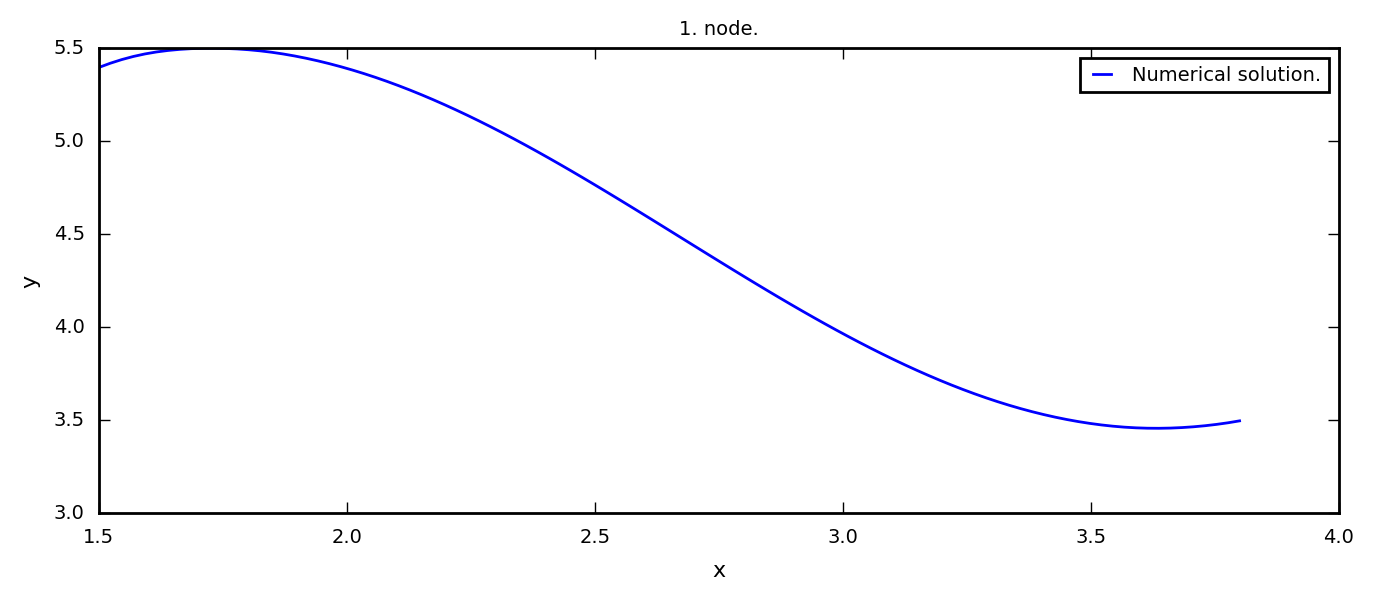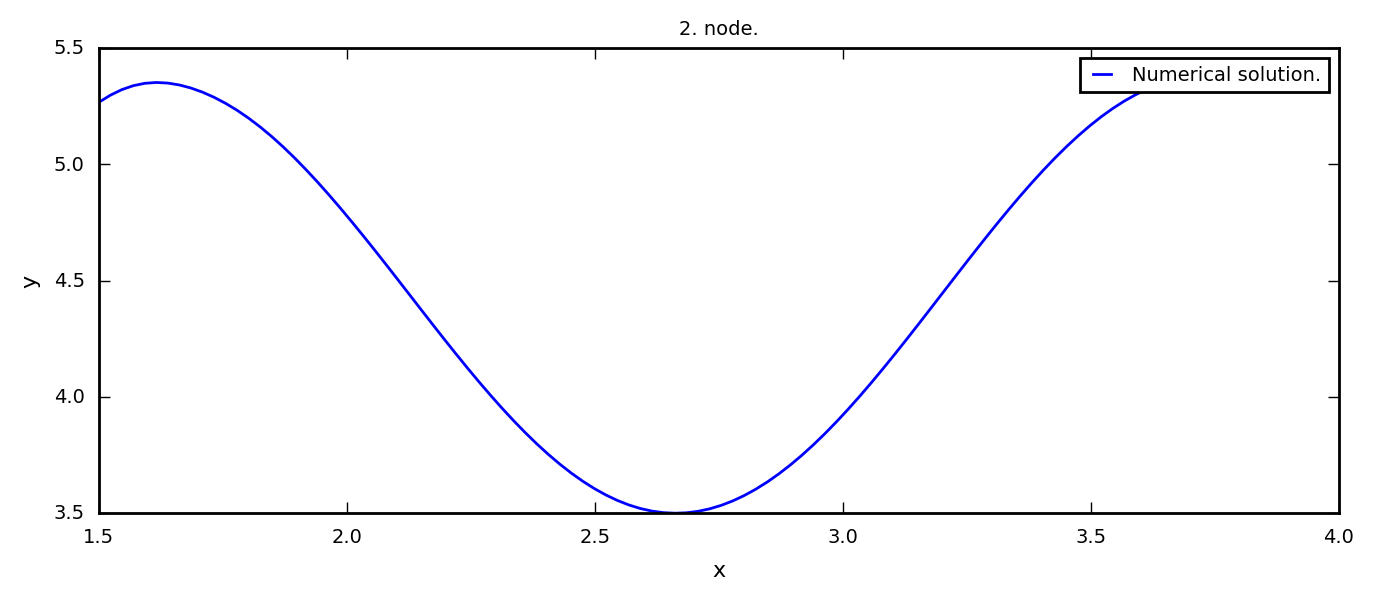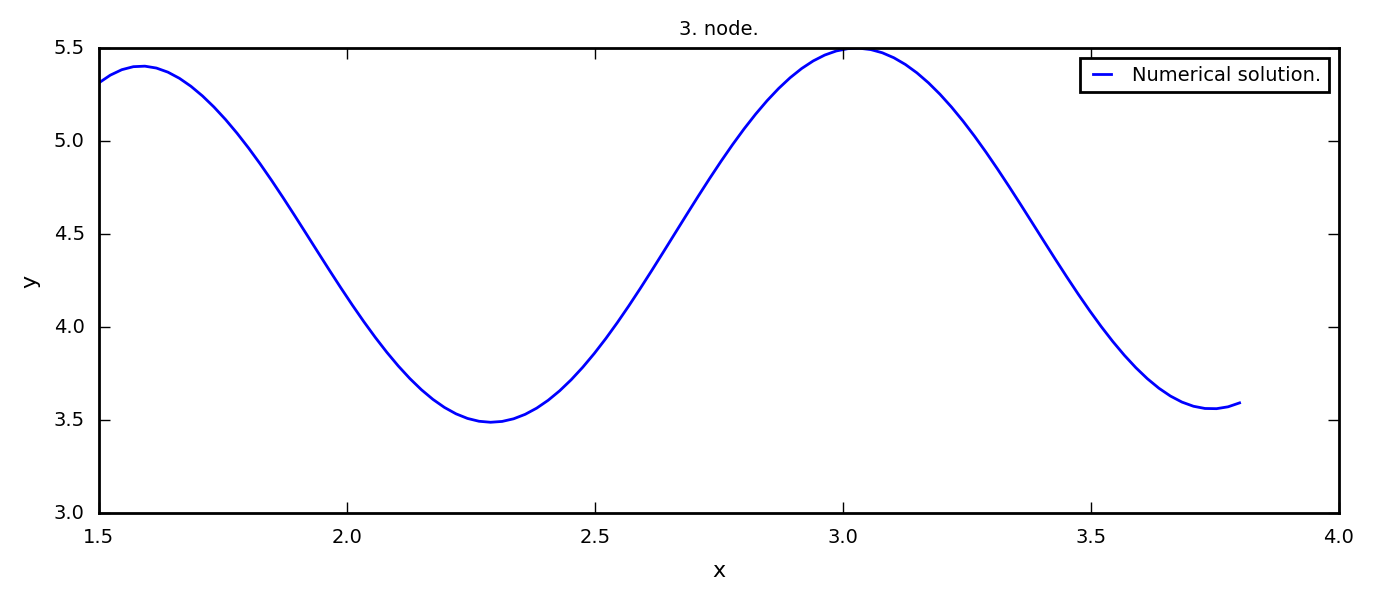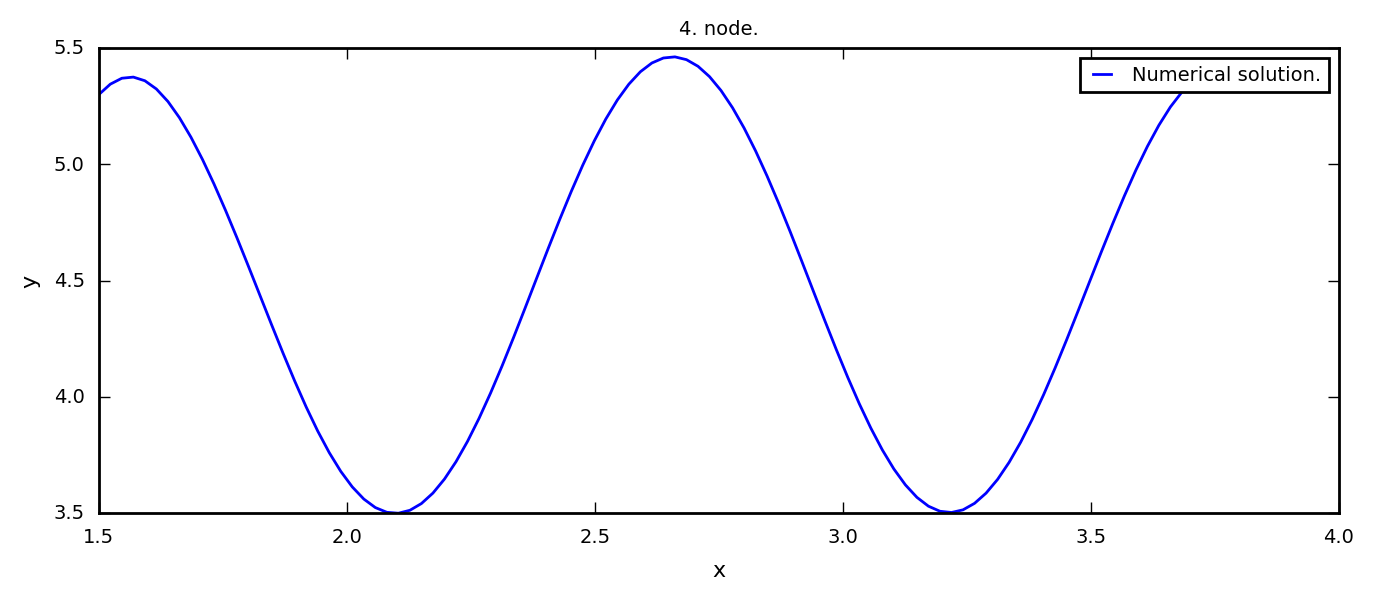#### 6 Some final notes.¶

An increased number of source and collocation points leads to an increased accuracy (try for yourself!). This is at the expense of increased computation time, since this leads to (amongst other) an increased number of equations in $A\boldsymbol \alpha = \lambda B \boldsymbol \alpha$. This also gives an increased number of valid eigenvalues; more eigenvalues are calculated with a better approximation, such that more eigenvalues are considered finite.

An increased $\delta$-value leads to a better accuracy for the low-valued eigenvalues, but might raise the error of the higher-valued eigenvalues. For example, the relative error of the smallest eigenvalues of the box-geometry with $N=200$ and $\delta = 1$ is $1.000\%$, $0.000\%$, $0.000\%$ and $21.394\%$.

$N=100$ and $\delta = 0.5$ are chosen as a compromise of the above disscussions and at the same time yield an acceptable error. Since the circular geometry and the general geometry are in the same order of magnitude as the box geometry, it is assumed that the same $N$ and $\delta$ yields good approximations for these geometries too. One can also check our calculations by increasing $N$ (or $\delta$) carefully, and check if it seems like the numerical values converges.

A potential conlcusion of which container is the best has to be based upon discussions about high points, amplitudes and eigenfrequencies/resonace frequencies. Moreover, the forces on the fluid and on the container (especially on large containers) should also be considered. The lowest eigenvalues are of most physical interest, since the approximations explained in the appendices are most accurate here.

Let us define the axes to have the unit m (meter). Then the eigenvalue has the unit m$^{-1}$, and we can use $g=9.81$ m/s$^2$. From the appendices we can see that $\lambda = \omega^2/g$. For example, the first node of the box geometry has in our calculations an eigenvalue $\lambda_1 = 1.016$ m$^{-1}$. This corresponds to a resonance frequency $f = \omega/2\pi = \sqrt{\lambda g}/2\pi \approx 0.502$ s$^{-1}$. Let us perform a more trivial example. Assume that an ordinary coffee cup is cylindric with a diameter of 6 cm and a height 10 cm. Our numerical calculations is done in two dimensions, but it would be reasonable to suppose that the eigenfrequencies of this cylinder would have eigenvalues in the same order of magnitude as a two dimensional box geometry of width 6 cm and height 10 cm. This box has an eigenvalue $\lambda_1 = 44.869$, which corresponds to the resonance frequency $f=3.339$ s$^{-1}$. Be careful about how fast you walk with your coffee mug!

# A Derivation of the equations.¶

## A.1 Partial differential equations in the fluid.¶

Assume that the fluid is ideal. This means that it is inkompressible, has zero viscocity and has a constant and uniform density distribution. The Euler equations for the fluid is then $$\sum_j \partial_j u_j = 0, \quad [\text{inkompressibility}]$$ $$\partial_t u_i + \sum_j u_j \partial_j u_i + \frac{1}{\rho}\partial_i p = a_i, \quad [\text{Newtons second law}]$$ where $\boldsymbol u = [u_1,u_2,u_3]: \Omega \times \mathbb R\to \mathbb R$ is the velocity, $p:\Omega\times \mathbb R\to\mathbb R$ is the pressure, and $\boldsymbol a = [a_1,a_2,a_3]:\Omega \times\mathbb R\to\mathbb R$ the remote acceleration (e.g. the gravitational acceleration). Assume also that $\partial_i u_j - \partial_ju_i = 0$ for all $i,j = 1,2,3$ (the assumption for potential flow). The velocity can then be written as $u_i=\partial_i\psi$ for a function $\phi:\omega \times \mathbb R \to \mathbb R$ (Hodge decomposition), then we get $$\sum_j \partial_j ^2\phi = 0, \quad[\text{Poisson's equation}]$$ $$\partial_i\partial_t\psi + \sum_j\partial_j\psi\partial_j\partial_i\psi + \frac{1}{\rho}\partial_ip = a_i.$$ If we assume $a_1=a_2=0$ and $a_3=-g$ (gravitational acceleration), then the last equation can be integrated to get Bernoulli's equation, which defines $p$ given $\psi$ $$\partial_t\psi + \frac{1}{2}\sum_j [\partial_j\psi]^2+\frac{p-p_\text{ref}}{\rho}+gx_3 = 0,$$ where $p_\text{ref}$ is a constant reference pressure (integration constant).

## A.2 Boundary conditions.¶

At the boundary we know that $\sum_jn_ju_j=V,$ where $V$ is the perpendicular velocity at the boundary. In other words, at the fixed container $\Gamma_\text B$ $$\sum_j n_j\partial_j \phi = 0,\quad (x_1,x_2,x_3)\in \Gamma_\text B.$$ The free surface is $(x_1,x_2,H+h(x_1,x_2,t))$, for an unknown function $h(x_1,x_2,t)$. We know that the pressure on the free surface is equal to the atmospheric pressure $p_\text{atm}$, and at $x_3=0$ the pressure is $p=p_\text{atm} + \rho g H$. We therefore insert $p_\text{ref}=p_\text{atm}+\rho g H$ into the last equation of A.1 and get $$\partial_t \phi + \frac{1}{2}\sum_j [\partial_j\phi]^2+gh = 0,$$ when $x_3 = H+h(x_1,x_2,t)$. Note that the amplitude is a function of $x_1$, $x_2$ and $t$: $x_3 = x_3(x_1,x_2,t)$. If we derivate $x_3 = H+h(x_1,x_2,t)$ with respect to $t$ and uses the chain rule, we get $$u_3 = \frac{\text dx_3}{\text dt} = \frac{\text dh}{\text dt} = \partial_th+u_1\partial_1h+u_2\partial_2h.$$ If we use the fact that $\boldsymbol u = \nabla \phi$, we get $$\partial_3 \phi = \partial_t h + \partial_1\phi\partial_1 h+\partial_2\phi\partial_2 h.$$